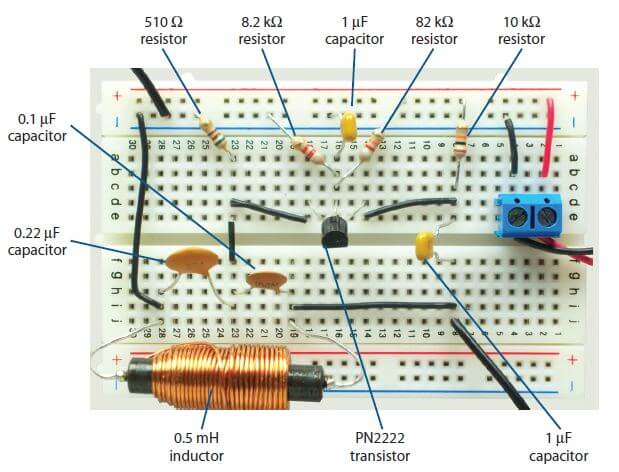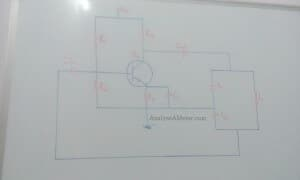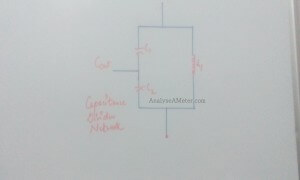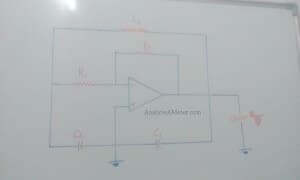# Colpitts oscillator: Working and Design using opamp

Colpitts oscillator is another type of oscillator design used to produce an oscillation at a certain frequency. It was invented by the American engineer Edwin H. Colpitts in 1918. In our previous articles, we explain about Oscillator circuit and Hartley oscillator in detail. In this tutorial, we are going to explain Colpitts oscillator circuit, basics in brief.It is designed for the generation of high-frequency sinusoidal oscillations with the radio frequencies typically ranging from 10 KHz to 300 MHz. The main distinguish feature of  Colpitts oscillator is that the it take feedback from the active device from the voltage divider made of two capacitors in series across the inductor.

# Colpitts oscillator circuit:

As shown in the figure below, the emitter terminal of the transistor is effectively connected across the junction of two capacitors C1 and C2. Resistors R1 and R2 gives the voltage divider biasing to the transistor, R4 limits the collector current of the transistor, Re is the emitter resistor used for maintaining thermal stability of the transistor. Cin and Cout are the input and output Dc decoupling capacitor, Ce is the emitter by-pass capacitor used to by-pass the amplified Ac signal from dropping across Re.Colpitts oscillator circuit diagram

As shown in the figure above, when we supply power across the circuit the capacitors C1 and C2 starts charging. Once the capacitors are fully charged they start discharging through the inductor L1. The electrostatic energy stored in the capacitors gets transferred to the inductor as a magnetic flux when the capacitors are fully discharged. Once the energy transferred, the inductors start discharging and capacitors get charged again.  This transfer of energy between capacitor and inductor depends on the oscillation. The voltage across C2 is fed back to the transistor is in opposite phase to that of the voltage across C1. Across the collector and emitter of the transistor, the feedback signal at the base appears in the amplified form. In oscillator circuit, 180o phase change occurs in the voltage between the base and collector and 180o occur along the feedback loop. By combining the phase changes, the correct phase relationship of positive feedback for oscillations will be maintained.

## Colpitts Oscillator basics:

#### Colpitts oscillator Tank circuit:

In Colpitts oscillator tank circuit, the centre tapping is made at the junction of a capacitive voltage divider network. As shown in figure below, the two series capacitors C1 & C2 are connected in parallel to the single common inductor L. This combination of capacitor and inductor is known as tuned tank circuit with the conditions of oscillations being Xc1 + Xc2 = XL same as for the Hartley oscillator circuit. The voltage across C2 is applied to the base-emitter junction of the transistor as feedback to create oscillations.Colpitts oscillator Tank circuit

The basic configuration difference between the Colpitts and Hartley oscillator is of centre tapping i.e. in Hartley oscillator centre tapping is done across the inductor voltage divider network while in Colpitts oscillator it is done across the Capacitive voltage divider network. In this type of circuit configuration, frequency stability of the oscillator is improved along with a more simple design because of the less self and mutual induction occurrence within the tank circuit.

#### Frequency of Colpitts oscillator:

The frequency of a Colpitts oscillator can be expressed using the equation:

F = 1 / 2∏ √ LC

Where

C = C1 C2 / (C1 + C2) i.e. capacitance of the capacitor in the tank circuit

L = Inductance of the inductor in the tank circuit

Note: Colpitt oscillator can be made variable by using the ganged variable capacitors in place of C1 and C2.

In this type of oscillator, the frequency of oscillations is slightly lower due to junction capacitances and resistive loading of the transistor. It can also be used as variable frequency oscillator and performs best when a variable inductance is used for tuning.

### Colpitts oscillator circuit using op-amp:

Colpitts oscillator circuit with operational amplifier generally facilitates the gain adjustment using the feedback resistance and internal resistance.Colpitts oscillator using op-amp

The typical arrangement is shown above indicates that in transistorized oscillator the gain depends on the tank circuit element Rf & R1 can be expressed by the equation A = -Rf / R1 and hence provide great frequency stability. The main advantage of using op amp is that the gain of the oscillator can be individually adjusted using the feedback resistor (Rf) and input resistor (R1). In colpitt oscillator, other components such as tank circuit elements, coupling capacitors etc have no significant effect on the gain of an op amp. The working principle and frequency equation of the op amp type is same as that of the transistor type.

#### Colpitts oscillator applications:

Following of the applications of Colpitts oscillator are:

• Using SAW device it can be used as a different type of sensors such as temperature sensor.
• It is used for the development of mobile and radio communication.
• With very high frequencies it is used for generating sinusoidal output signals.
• It is used for commercial purposes.
• It is used for applications in which undamped and continuous oscillations are desired for functioning.
• It can be used in microwave applications.

Hope you all like this article. for any suggestions please comment below. We always appreciate your suggestions.

Like this? Vote now
[Total: 0 Average: 0]

### 3 thoughts on “Colpitts oscillator: Working and Design using opamp”

This site uses Akismet to reduce spam. Learn how your comment data is processed.

error: Stop Stealing content, do some hardwork !!